Updated by Laramy Wells on Feb 21, 2014REPORTLaramy Wells
Owner
15 items   3 followers   0 votes   531 views

# Factoring Polynomials

The following is a collection of websites, videos, and such that will help you with factoring polynomials. Most resources are specific to quadratics and cover everything from greatest common factor (GCF) to factoring trinomials with a>1.

1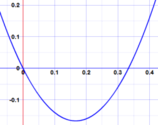To "Factor" (or "Factorise" in the UK) a Quadratic is to: find what to multiply to get the Quadratic It is called "Factoring" because we find the factors (a factor is something we multiply by) Example The factors of are: Why?

2This Khan Academy video goes through the detailed process of factoring. The instructor breaks down the process in a very rigorous manner. Khan Academy videos do require previous background knowledge and you may need to review previous videos in this series.

3

## Factoring Monomials from a PolynomialThe following video is from Mr. Tarrou (ProfRobBob) on YouTube. This guys takes a very enthusiastic look at mathematics. This video deals with the first step to consider when factoring a polynomial is to check for a Greatest Common Factor (GCF). This video reviews the process of factoring out a monomial from a polynomial

4

## Factoring Polynomials: Factoring by Grouping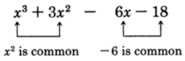Problems that feature an even number of terms can be factored by grouping. Factoring by grouping does require knowing how to factor monomials from a polynomial. This process can also be used in some methods to factor trinomials even though they have an odd number of terms. The website below gives detailed explanations and examples for the process.

5

## Factoring Quadratic Trinomial x^2+bx+c PolynomialWhen dealing with quadratic trinomials with a leading coefficient of 1, the process is quite simple. Mr. Tarrou demonstrates basic factoring and goes through the patterns of the signs.

6

## Factoring SignsHere is a simple graphic that explains the process of knowing which signs to use in your factorization.

7Mr. Tarrou demonstrates factoring for quadratic trinomials with leading coefficients that do not equal 1. He will utilize the process of factoring by grouping.

8

## Special Factoring: Differences of SquaresAlthough the basics for factoring will hold true in every case, there are some special factoring problems to take note of in a problem. One special case is the difference of squares. Purplemath gives a wonderful explanation of this process that you must consider whenever you are missing the "b" term of your quadratic.

9

## Factoring Perfect Square Trinomials - MathBitsNotebook(A1 - CCSS Math)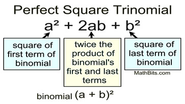Another special case is the perfect square trinomial. Though harder to visually identify, this site gives a breakdown of how to read a trinomial to determine if it is in fact a perfect square.

10

## Factoring Flowchart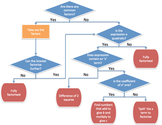Here is a TERRIFIC flowchart to follow so that you will know what you need to do with a factoring problem.

11

## Free Online Quadratic Equation Solver: Solve by Factoring (or Factoring by Grouping)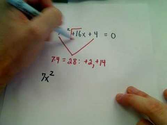Though I am against most automatic equation calculators online, this one is different. Not only will it factor and solve your quadratic equation, but it will provide a step-by-step explanation of the process. This way you can still learn the material for your specific problem.

12

## Factoring when a=1This is an image from a student's interactive notebook with the process for factoring a quadratic trinomial that has a leading coefficient of 1. This demonstrates the X-Factor or "Criss-Cross" method.

13

## Factoring when |a|>1Here is an image from a student's interactive notebook with the process for factoring a quadratic trinomial that has a leading coefficient not equal to 1. This demonstrates the X-Factor or "Criss-Cross" method with the "Slide and Divide" process.

14

## Twitter / FlowersMath: Just in case you need help! ...Newton High School math teacher Ms. Flowers created this guide for her students and tweeted it out for additional help with factoring.

15

## Factoring Trinomials Tic-Tac-Toe Method for You Tube.wmv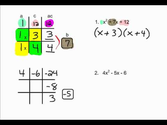People seem to create different methods for factoring all the time. Below is a video of a fairly new method called the tic-tac-toe method. This method puts the information into a visual representation that may benefit those who struggle with the box method or X-factor.